Fractal Art Gallery Equation Studies Home Art Math Business

Here are two different equation studies: Two series of somewhat pronouncedly
diverse images with given constants (when applicable)...

 f(z) = z^3 + c f(z) = (z^7 + 1)^(1/3) + c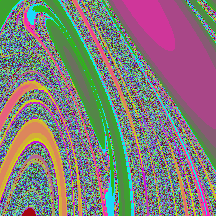c = .406775 + .227114 i c = .406 + .227 i Mandelbrot set, no c Mandelbrot set, no c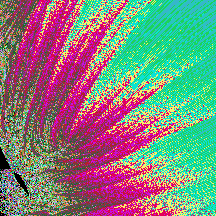Mandelbrot set; no c c = .36 + .45 i Mandelbrot set, no c Mandelbrot set, no c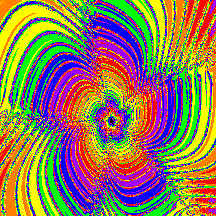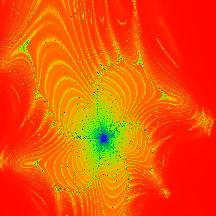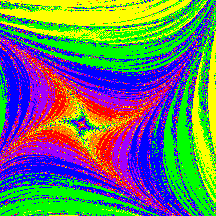c = .2525 + .63 i c = .2525 + .63 i c = - .32 - .857 i c = - .32 - .857 i